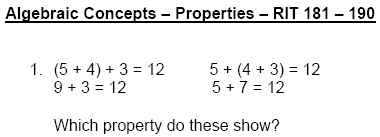Name:    3rd Grade Basic Math Test 1

Multiple Choice
Identify the choice that best completes the statement or answers the question.

1.

Algebraic Concepts – Solving Equations & Inequalities – RIT 161 – 170
7 + ___ = 9
 a. 3 d. 1 b. 2 e. 5 c. 4

2.

Ten cars were in a race.  Two cars did not finish.  How would you find the number of cars that did finish.
 a. add (+) d. divide () b. subtract (-) e. none of the above c. multiply (x)

3.

Algebraic Concepts – Properties – RIT 171 – 180

2 x 3 = 3 x ___
 a. 6 d. 2 b. 5 e. 9 c. 3

4.

Algebraic Concepts – Solving Equations & Inequalities – RIT 171 – 180
Find the missing factor.

3 x ___ = 15
 a. 4 d. 12 b. 5 e. 7 c. 6

5.

6 + 3 - 4 = ____
 a. 13 d. 5 b. 7 e. 10 c. 9

6.

Algebraic Concepts – Ordered Pairs – RIT 181 – 190a. Point A d. Point D b. Point B e. Point E c. Point C

7.

Algebraic Concepts - Patterns, Sequences, Functions - RIT 181 - 190

1,  4,  7,  10,  ___ , ____

What are the next two numbers in the pattern?
 a. 12, 14 d. 13, 17 b. 14, 15 e. 13, 16 c. 11, 12

8.

10  8  ?  4  2
What is the missing number in this pattern?
 a. 6 d. 3 b. 9 e. 7 c. 5

9.a. 4 d. 10 b. 5 e. 8 c. 12

10.a. Associative Property d. Addition Property b. Commutative Property e. Subtraction Property c. Zero Property

11.

Algebraic Concepts - Patterns, Sequences, Functions - RIT 181 - 190
If 6x = 12, what does x equal?
 a. 6 d. 5 b. 3 e. 12 c. 2

12.

5 + ____ = 7
 a. 3 d. 2 b. 57 e. 12 c. 75

13.

12 + _____ = 14
 a. 26 d. 12 b. 1 e. 2 c. 3

14.

Computation – Whole Numbers – RIT < 150
3 + 1 = 4
1 + 3 = 4
4 – 1 = 3
Which fact is missing?
 a. 4 – 3 = 1 d. 3 + 2 = 5 b. 4 + 3 = 7 e. 3 + 4 = 7 c. 4 +1 = 5

15.

5
- 2
 a. 5 d. 7 b. 3 e. 6 c. 2

16.

l  l  l  l   +   l l l = _____
 a. 5 d. 4 b. 3 e. 6 c. 7

17.

3
1
+ 4
 a. 7 d. 8 b. 5 e. 4 c. 9

18.

125
+ 43
 a. 179 d. 199 b. 168 e. 158 c. 62

19.

12
- 5
 a. 5 d. 7 b. 8 e. 17 c. 6

20.

X    X           X    X           X    X
X                  X

X

3 x 3 =
 a. 9 d. 3 b. 6 e. 8 c. 12